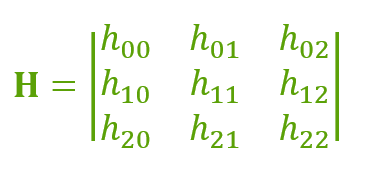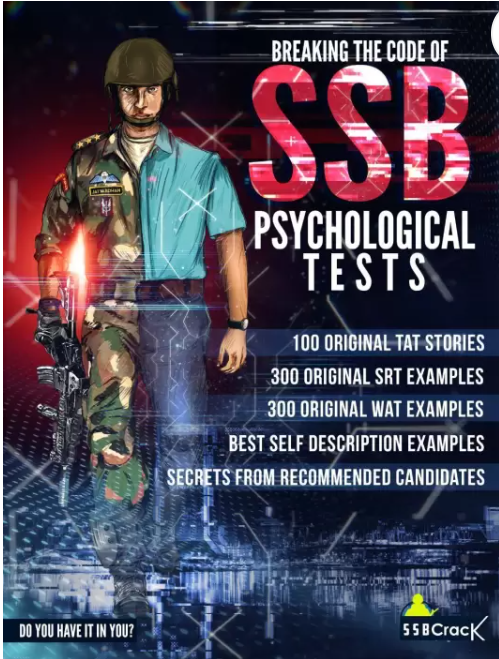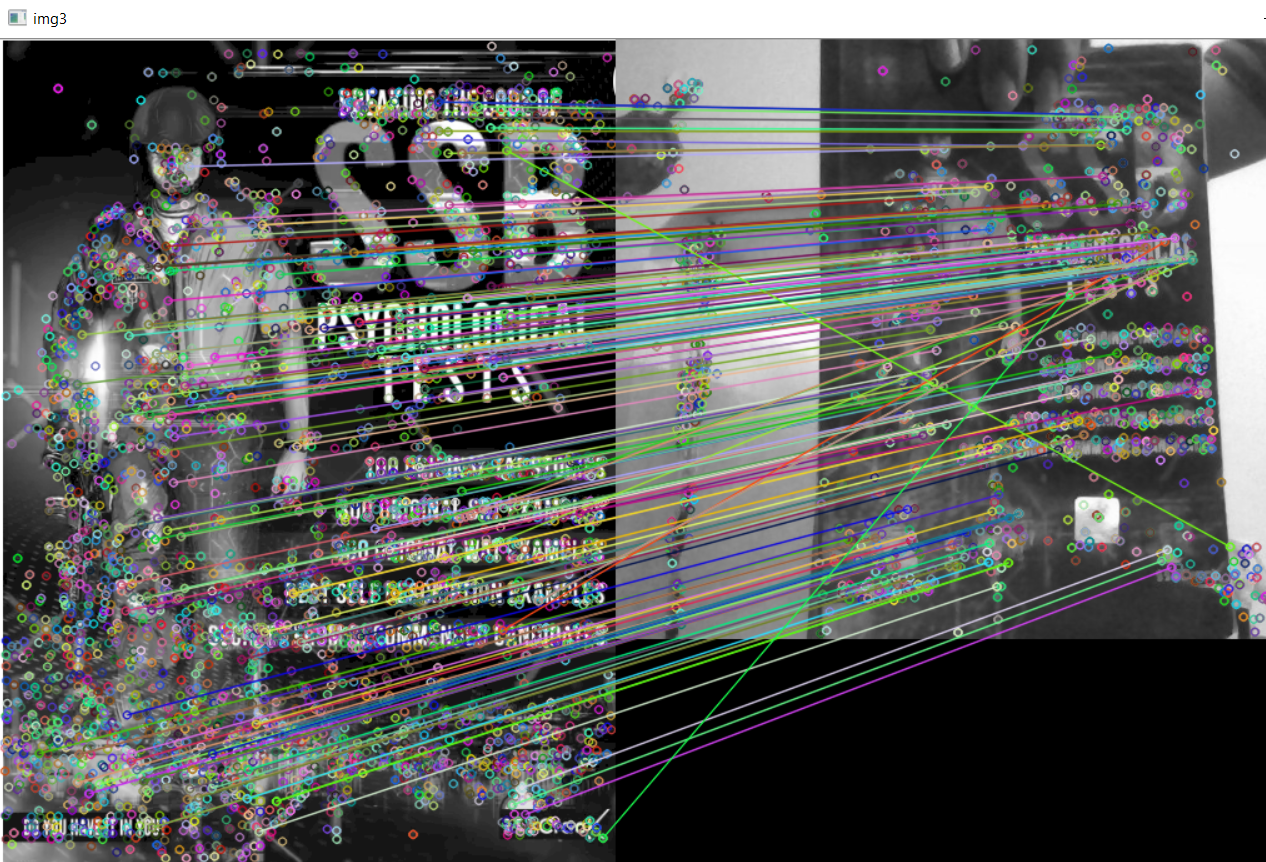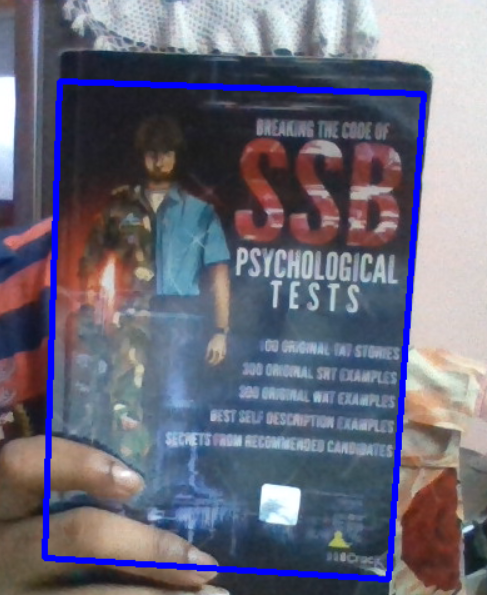Related Articles

# Python OpenCV: Object Tracking using Homography

• Difficulty Level : Hard
• Last Updated : 13 Sep, 2021

In this article, we are trying to track an object in the video with the image already given in it. We can also track the object in the image. Before seeing object tracking using homography let us know some basics.

## What is Homography?

Homography is a transformation that maps the points in one point to the corresponding point in another image. The homography is a 3×3 matrix :If 2 points are not in the same plane then we have to use 2 homographs. Similarly, for n planes, we have to use n homographs. If we have more homographs then we need to handle all of them properly. So that is why we use feature matching.

Importing Image Data : We will be reading the following image :Above image is the cover page of book and it is stored as ‘img.jpg’.

## Python

 `# importing the required libraries``import` `cv2``import` `numpy as np` `# reading image in grayscale``img ``=` `cv2.imread(``"img.jpg"``, cv2.IMREAD_GRAYSCALE)` `# initializing web cam``cap ``=` `cv2.VideoCapture(``0``)`

Feature Matching : Feature matching means finding corresponding features from two similar datasets based on a search distance. Now will be using sift algorithm and flann type feature matching.

## Python

 `# creating the SIFT algorithm``sift ``=` `cv2.xfeatures2d.SIFT_create()` `# find the keypoints and descriptors with SIFT``kp_image, desc_image ``=``sift.detectAndCompute(img, ``None``)` `# initializing the dictionary``index_params ``=` `dict``(algorithm ``=` `0``, trees ``=` `5``)``search_params ``=` `dict``()` `# by using Flann Matcher``flann ``=` `cv2.FlannBasedMatcher(index_params, search_params)`

Now, we also have to convert the video capture into grayscale and by using appropriate matcher we have to match the points from image to the frame.

Here, we may face exceptions when we draw matches because infinitely there will we many points on both planes. To handle such conditions we should consider only some points, to get some accurate points we can vary the distance barrier.

## Python

 `# reading the frame``_, frame ``=` `cap.read()` `# converting the frame into grayscale``grayframe ``=` `cv2.cvtColor(frame, cv2.COLOR_BGR2GRAY)` `# find the keypoints and descriptors with SIFT``kp_grayframe, desc_grayframe ``=` `sift.detectAndCompute(grayframe, ``None``)` `# finding nearest match with KNN algorithm``matches``=` `flann.knnMatch(desc_image, desc_grayframe, k``=``2``)` `# initialize list to keep track of only good points``good_points``=``[]` `for` `m, n ``in` `matches:``    ``#append the points according``    ``#to distance of descriptors``    ``if``(m.distance < ``0.6``*``n.distance):``        ``good_points.append(m)`Homography : To detect the homography of the object we have to obtain the matrix and use function findHomography() to obtain the homograph of the object.

## Python

 `# maintaining list of index of descriptors``# in query descriptors``query_pts ``=` `np.float32([kp_image[m.queryIdx]``                 ``.pt ``for` `m ``in` `good_points]).reshape(``-``1``, ``1``, ``2``)` `# maintaining list of index of descriptors``# in train descriptors``train_pts ``=` `np.float32([kp_grayframe[m.trainIdx]``                 ``.pt ``for` `m ``in` `good_points]).reshape(``-``1``, ``1``, ``2``)` `# finding  perspective transformation``# between two planes``matrix, mask ``=` `cv2.findHomography(query_pts, train_pts, cv2.RANSAC, ``5.0``)` `# ravel function returns``# contiguous flattened array``matches_mask ``=` `mask.ravel().tolist()`

Everything is done till now, but when we try to change or move the object in another direction then the computer cannot able to find its homograph to deal with this we have to use perspective transform. For example, humans can see near objects larger than far objects, here perspective is changing. This is called Perspective transform.

## Python

 `# initializing height and width of the image``h, w ``=` `img.shape` `# saving all points in pts``pts ``=` `np.float32([[``0``, ``0``], [``0``, h], [w, h], [w, ``0``]])``            ``.reshape(``-``1``, ``1``, ``2``)` `# applying perspective algorithm``dst ``=` `cv2.perspectiveTransform(pts, matrix)`

At the end, lets see the output

## Python

 `# using drawing function for the frame``homography ``=` `cv2.polylines(frame, [np.int32(dst)], ``True``, (``255``, ``0``, ``0``), ``3``)` `# showing the final output``# with homography``cv2.imshow(``"Homography"``, homography)`

Output :Attention geek! Strengthen your foundations with the Python Programming Foundation Course and learn the basics.

To begin with, your interview preparations Enhance your Data Structures concepts with the Python DS Course. And to begin with your Machine Learning Journey, join the Machine Learning – Basic Level Course

My Personal Notes arrow_drop_up